# 折线图

import matplotlib.pyplot as plt
import pandas as pd
import pymysql

#建立数据库连接
conn = pymysql.connect("192.168.6.128","root","root","test_db",charset="utf8")
print("连接成功")
#读取数据库表数据
data = pd.read_sql("select CAST(SUBSTRING(DT_DATE,9,2) AS SIGNED) DT_DATE,HIGH_TEMP,LOW_TEMP from tb",con=conn)
#数据转化为列表
x = list(data.DT_DATE) #日期
y = list(data.HIGH_TEMP) #最高气温
z = list(data.LOW_TEMP) #最低气温

#设置折线样式
plt.plot(x,y,"g--")
plt.plot(x,z,"r--")

#设置x坐标轴的范围
plt.xlim(1,30)
#设置y坐标轴的范围
plt.ylim(-50,50)

#设置X轴文字的标题
plt.xlabel("date")
#设置Y轴文字的标题
plt.ylabel("temperature(℃)")

#设置图表的标题
plt.title("Kunming temperature change chart in February")

plt.show()
print(type(x))
#关闭数据库连接
conn.close()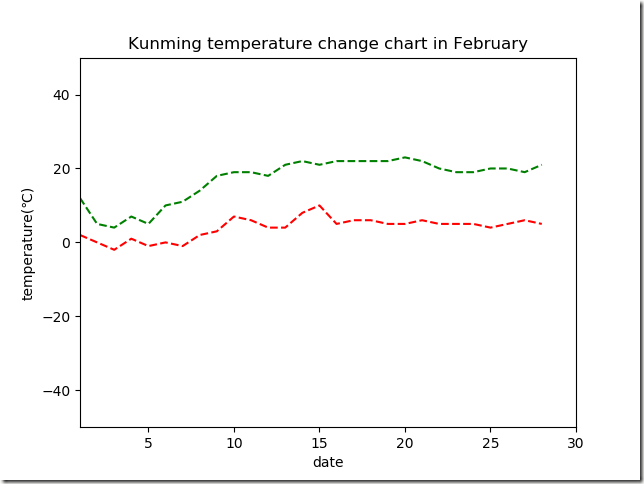## 设置线条样式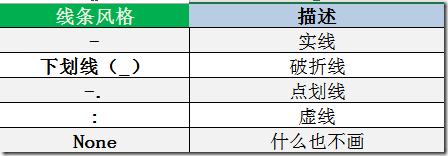## 设置线条的标记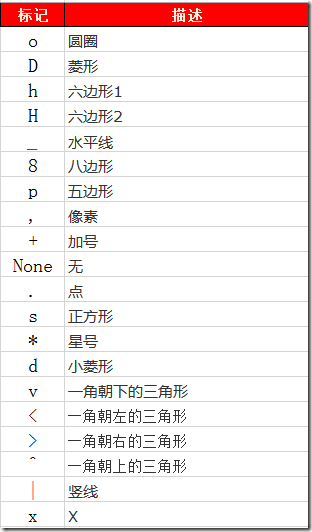## 线条的颜色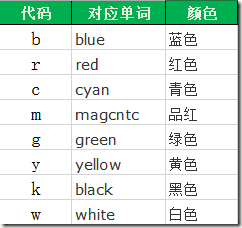# 散点图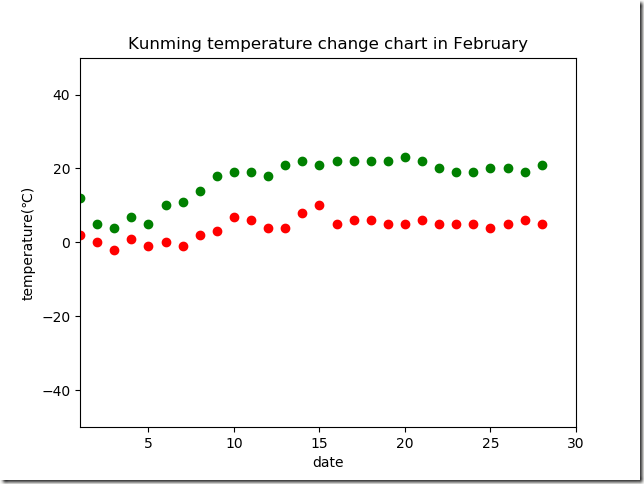posted @ 2018-05-01 23:12  OLIVER_QIN  阅读(12148)  评论(0编辑  收藏  举报